﻿ Finite Difference Technique for Unsteady MHD Periodic Flow of Viscous Fluid through a Planer Channel

### Finite Difference Technique for Unsteady MHD Periodic Flow of Viscous Fluid through a Planer Channel

Pawan Preet Kaur, SP Agrawal, Anil KumarOPEN ACCESSPEER-REVIEWED

## Finite Difference Technique for Unsteady MHD Periodic Flow of Viscous Fluid through a Planer Channel

Pawan Preet Kaur1, SP Agrawal2, Anil Kumar3,1Deparment of Applied Mathematics, Lyallpur Khalsa College Engineering Jalandhar Punjab

2Department of Civil Engineering, World Institute of Technology Sohna, Gurgaon, India

3Department of Applied Mathematics, World Institute of Technology Sohna, Gurgaon, India

### Abstract

In the present paper we investigate an unsteady periodic flow of a viscous incompressible fluid through a porous planer channel in the presence of transverse magnetic field. The governing equations have been solved by finite difference method. The analytical expressions for the velocity profiles of the fluid have been obtained and numerical computations involved in the solution have been shown by tables. The effects of various parameters on the flow field have been discussed with the help of graphs and tables.

### At a glance: Figures

123
Prev Next

• Kaur, Pawan Preet, SP Agrawal, and Anil Kumar. "Finite Difference Technique for Unsteady MHD Periodic Flow of Viscous Fluid through a Planer Channel." American Journal of Modeling and Optimization 1.3 (2013): 47-55.
• Kaur, P. P. , Agrawal, S. , & Kumar, A. (2013). Finite Difference Technique for Unsteady MHD Periodic Flow of Viscous Fluid through a Planer Channel. American Journal of Modeling and Optimization, 1(3), 47-55.
• Kaur, Pawan Preet, SP Agrawal, and Anil Kumar. "Finite Difference Technique for Unsteady MHD Periodic Flow of Viscous Fluid through a Planer Channel." American Journal of Modeling and Optimization 1, no. 3 (2013): 47-55.

 Import into BibTeX Import into EndNote Import into RefMan Import into RefWorks

### 1. Introduction

The fluid flow through porous medium has been of great interest for a long time. In the history of fluid flow through channels, Navier investigated a boundary condition of fluid slip at solid surface such that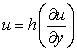, where h is the slip coefficient and u the velocity along x axis. In a case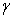=0 indicates that there is no slip at the boundary. Sparrow and Cess (1961) investigated the effect of a magnetic field on a free convective heat transfer. Raptis et al. (1982) have been discussed hydro magnetic free convection flow through a porous medium between two parallel plates. Pal et al. (1984) investigated a longitudinal dispersion of tracer particles in a channel bounded by porous media using slip condition. Singh and Kumar (1993) investigated a free convective fluctuating flow through a porous medium with variable permeability. Aldoss et al. (1995) depicted a mixed convection flow from a vertical plate embedded in porous medium in the presence of magnetic field. Makinde (1995) investigated a laminar flow in a channel of varying width with permeable boundaries. Soltani and Yilmazar (1998) studied a slip velocity and slip layer thickness in flow of concentrated suspensions. Jha (1998) discussed an effect of applied magnetic field on transient free convective flow in a vertical channel.

Helmy (1998) investigated MHD unsteady free convective flow past a vertical porous plate.Hossain et al. (1998) investigated heat transfer response of MHD free convective flow along a vertical plate to surface temperature oscillation. Kim (2000) discussed an unsteady MHD convective heat transfer past a semi-infinite vertical porous moving plate with variable suction. Yu and Ameel (2002) investigated a slip low heat transfer in rectangular micro channels. Derek et al. (2002) established an apparent fluid slip at hydrophibic microchannel walls. Sharma et al. (2002) investigated the flow between annular spaces surrounded by coaxial cylindrical porous medium. Steinruck (2003) investigated about the physical relevance of similarity solution of the boundary layer flow equation describing mixed convection flow along a vertical plate. Khaled and Vafai (2004) discussed the effect of slip condition on Stokes and Coutte flows due to an oscillating wall. Makinde and Mhone (2005) investigated a heat transfer to MHD Oscillatory flow in a channel filled with porous medium. Makinde and Osalusi (2006) discussed a MHD steady flow in a channel with slip at permeable boundaries. Kumar et al. (2007) investigated a viscous flow coaxial cylinder in the presence of magnetic field. Mahmood and Ali (2007) established the effect of slip condition on unsteady MHD oscillatory flow of a viscous fluid in a planer channel. Mishra et al. (2008) investigated a flow and heat transfer of a MHD viscoelastic fluid in a channel with stretching walls. Kumar et al. (2010) investigated Finite difference technique for reliable MHD steady flow through channels permeable boundaries. Kumar et al. (2013) investigated a Finite difference technique for reliable MHD steady flow through channels permeable boundaries. Kumar et al. (2013) made a Perturbation Technique of MHD Free Convection Flow through Infinite Vertical Porous Plate with Constant Heat Flux. Kumar and Agrawal (2013) profounded Mathematical and Simulation of lid driven cavity flow at different aspect ratios using single relaxation time lattice Boltzmann technique. Kumar et al. (2013) investigated Finite Element Galerkin’s Approach for Viscous Incompressible Fluid Flow through a Porous Medium in Coaxial Cylinders.

### 2. Formulation of the Problem

We are considering an unsteady periodic flow of viscous incompressible fluid through a planer channel with saturated porous medium in the presence of transverse magnetic field. The governing equations of the motion are given below: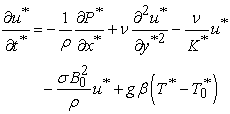(1)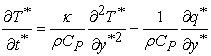(2)

Boundary conditions are: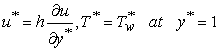(3)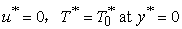(4)

where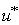is the velocity,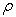the fluid density,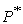the pressure,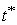the time,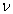the kinematics viscosity coefficient,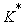the porous medium permeability coefficient,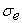the conductivity of the fluid,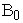the electromagnetic induction, g the acceleration due to gravity,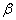the coefficient of volume expansion due to temperature,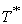the fluid temperature,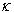the thermal conductivity,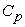the specific heat at constant pressure and q is the radiative heat flux. Consider the fluid is optically thin with a relatively low density and the radiative heat flux is given by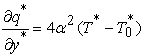(5)

Where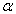is the mean radiation absorption coefficient,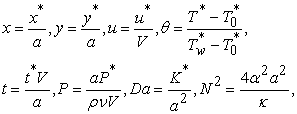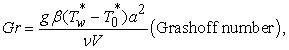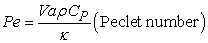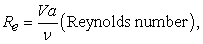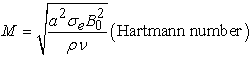where V is the mean of flow velocity

Using the above dimensionless quantities into equations (1) to (4), we have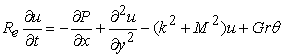(6)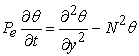(7)

Subject to condition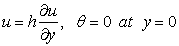(8)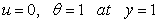(9)

where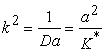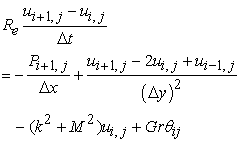(10)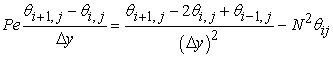(11)

The corresponding boundary conditions are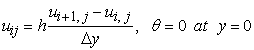(12)(13)

The system of algebraic equations can be solved by Gauss-Siedal method or any other similar method. We use the Thomas algorithm.

The shearing stress is given by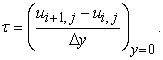### 3. Computational Algorithm

We solve these coupled non linear partial differential equations by standard Finite difference technique [Kumar et al. (2013)]. The spetially discrete form of the governing equations can be obtained by Finite difference technique. A computational iteration procedure for the equations (10)-(11) with the boundary conditions (12) and (13) has been followed which takes care of highly non linear coupled set of algebraic equations. The computational iteration process may be divided into the following steps:

Step 1. Simplify equation (10) for j = 2, 3,…n-1 and i = 2,3,….m-1. Calculate the velocity profile using boundary conditions (12) and (13).

Step 2. The algebraic equations are solved by using Guass-Seidal method

Step 3. Update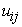from the previous step.

Step 4. Repeate steps (1)-(3) until convergence are reached.

### 4. Result and Discussion

The effects of various parameters like Hartmann number M, Grashoff number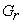, Peclet number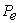, Reynolds number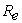, permeability of porous medium k, Radiation parameter N, time t and wall slip parameter h on velocity and temperature profiles have been shown in tables and graphs. The brief description is as given below:

#### Table 1. Velocity profiles for different values of parameter

Figure 1. Velocity profiles for various values of wall slip parameter h

#### Table 2. Shear stress for different values of parameter

Figure 1 shows that the velocity is everywhere increases corresponding to every increase in the wall slip parameter h in the region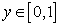, which clearly tallies the natural phenomenon.

Figure 2 depicts the tendency of shear stress distribution corresponding to different values of wall slip parameter h. Hence for every smaller values of h the value of shear stress is seen much more than that of larger one.

#### Table 3. Velocity profiles for different values of parameter

Figure 3 depicts that the velocity profile of the fluid is periodic with period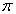.

Figure 4 depicts velocity profile for various values of magnetic parameter M. It shows that the flow decelerates on the imposition of magnetic field i.e. on the increase of magnetic parameter M.

#### Table 5. Velocity profiles for different values of parameter

Figure 5 depicts that velocity profile for different values of porosity parameter k.

The effect of Peclet numberon the velocity profiles are shown in Table 6 and Figure 6. It is found that the fluid velocity decreases as the values of Peclet number increases corresponding to the same value of y.

Table 7 & Figure 7 also show similar effects of Reynolds numberas the Peclet number has the flow.

#### Table 8. Velocity profiles for different values of parameter

Table 8 & Figure 8 depict the velocity profiles for various values of radiation parameter N. From Table 8 or Figure 8, it is observed that the increasing values of radiation parameter N have the similar effects on the velocity distribution as parameterand numberhave.

### Nomenclature: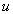: Velocity along x axis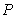: Fluid pressure: Fluid density: Kinematic viscosity: Electromagnetic induction: Fluid conductivity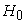: Intensity of magnetic field: Coefficient of volume expansion due to temperature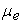: Magnetic permeability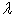: A constant: Specific heat at constant pressure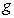: Acceleration due to gravity

M: Hartmann number: Thermal conductivity

K: Porous medium permeability

k: Porous medium shape factor

T: Fluid temperature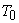: Temperature at the boundary y = 0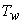: Temperature at the boundary y = a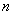: Frequency of the periodic flow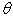: Non dimensional temperature: Reynolds number: Grashoff number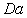: Darcy number

### References

  Sparrow, E.M. and Cess, R.D. (1961): The effect of a magnetic field on a free convective heat transfer, International Journal of Heat and Mass Transfer, 3, 267-274.In article CrossRef  Raptis, A.; Massias, C. and Tzivanidis, G. (1982): Hydromagnetic free convection flow through a porous medium between two parallel plates, Phys. Lett., 90(A), 288-289.In article  Pal, D.; Veerbhadraiah, R.; Shivakumar, P.N. and Rudraiah, N. (1984): Longitudinal dispersion of tracer particles in a channel bounded by porous media using slip condition, International Journal of Mathematical science, 7,755-764.In article  Singh, K.D. and Kumar, Suresh (1993): Free convective fluctuating flow through a porous medium with variable permeability, Journal of Mathematical and Physical Sciences, 27(2), 141-148.In article  Aldoss, T.K.; Al-Nimr, M.A.; Jarrah, M.A. and Al-Shaer, B.(1995): Mixed convection flow from a vertical plate embedded in porous medium in the presence of magnetic field, Heat Transfer, 28(A), 635-645.In article  Malcinde, O.D. (1995): Laminar flow in a channel of varying width with permeable boundaries, Romanian Journal of Physics, 40(4-5), 403.In article  Soltani, F. and Yilmazar, U. (1998): Slip velocity and slip layer thickness in flow of concentrated suspensions, Journal of Applied Polymer Science, 70, 515-522.In article CrossRef  Jha, B.K. (1998): Effect of applied magnetic field on transient free convective flow in a vertical channel, Indian Journal of Pure and Applied Mathematics, 29(4), 441-445.In article  Helmy, K.A. (1998): MHD unsteady free convective flow past a vertical porous plate, Z AMP, Z.Angew Math. Mech., 78(4), 255-270.In article CrossRef  Hossain, M.A.; Das, S.K. and Pop, I.(1998): Heat transfer response of MHD free convective flow along a vertical plate to surface temperature oscillation, International Journal of Non-Linear Mechanics, 33(3), 541-553.In article CrossRef  Kim, Y.J. (2000): Unsteady MHD convective heat transfer past a semi-infinite vertical porous moving plate with variable suction, International Journal of Engineering Science, 38,833-842.In article CrossRef  Yu, S. and Ameel, T.A. (2002): Slip low heat transfer in rectangular micro channels, International Journal of Heat and Mass Transfer, 44, 4225-4234.In article CrossRef  Derek, C.; Tretheway, D.C. and Mainhart, C.D. (2002): Apparent fluid slip at hydrophibic micro channel walls, Physics of Fluids, 14, L9-L12.In article CrossRef  Sharma, G.C.; Jain, M. and Kumar, Anil (2002): The flow between annular space surrounded by coaxial cylindrical porous medium, Published in National Conference on 67th Indian Mathematical Society, AMU, Aligarh, Jan. 27-30, 2002.In article  Steinruck, H. (2003): About the physical relevance of similarity solution of the boundary layer flow equation describing mixed convection flow along a vertical plate, Fluid Dynamics Research, 32, 1-13.In article CrossRef  Khaled, A.R.A and Vafai, K. (2004): The effect of slip condition on stokes and coutte flows due to an oscillating wall, International Journal of Nonlinear Mechanics, 39, 795-809.In article CrossRef  Makinde, O.D. and Mhone, P.Y. (2005): Heat transfer to MHD Oscillatory flow in a channel filled with porous medium, Romanian Journal of physics, 50(9-10), 931-938.In article  Makinde, O.D. and Osalusi, E. (2006): MHD steady flow in a channel with slip at permeable boundaries, Romanian Journal of Physics, 51(3-4), 319-328.In article  Kumar, Anil; Varshney, C.L. and Sajjan Lal (2007): Viscous flow coaxial cylinder in the presence of magnetic field, Published in the 15th International conference on Interdisciplinary Mathematical and Statistical Technique, Shanghai, China, May20-23.In article  Mahmood, A. and Ali, A. (2007): The effect of slip condition on unsteady MHD Oscillatory flow of a viscous fluid in a planer channel, Romanian Journal of Physics, 52, Nos. 1-2, 85-91.In article  Mishra, J.P.; Shit, G.C. and Rath, H.J. (2008): Flow and heat transfer of a MHD visco-elastic fluid in a channel with stretching walls, Computers and Fluids, 37(1), 1-11.In article CrossRef  Anil Kumar, CL Varshney and Sajjan Lal (2013): Performance Modeling and analysis of Non-Viscous Incompressible Fluid Flow and Convective Temperature Flow in the Magnetic effects, International Journal of Mathematical Modeling and Physical Sciences Vol. 01, ( 01) pp 10-15, March 2013.In article  Anil Kumar and S P Agrawal (2013): Mathematical and Simulation of lid driven cavity flow at different aspect ratios using single relaxation time lattice Boltzmann technique, American Journal of theoretical and Applied Statistics, vol 2 (3), pp 87-93.In article  Anil Kumar , SP Agrawal and Pawan Preet Kaur (2013)Finite Element Galerkin’s Approach for Viscous Incompressible Fluid Flow through a Porous Medium in Coaxial Cylinders, Journal of Mathematical Sciences and Applications, 2013, Vol. 1, No. 3, 39-42.In article  Anil Kumar, CL Varshney and Sajjan Lal (2013): Perturbation Technique of MHD Free Convection Flow through Infinite Vertical Porous Plate with Constant Heat Flux, International Journal of Mathematical Modeling and Physical Sciences Vol. 01, (02) pp 1-5, 2013.In article CrossRef  Anil Kumar, R. K. Saket, C L Varshney and Sajjan Lal : Finite difference technique for reliable MHD steady flow through channels permeable boundaries, International Journal of Biomedical Engineering and Technology (IJBET) UK, Vol. 4(2) pp 101-110, 2010.In article